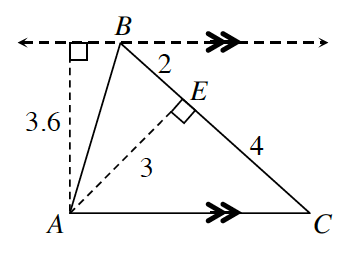### Home > CCG > Chapter 6 > Lesson 6.2.1 > Problem6-56

6-56.Penelope measured several sides and heights of $ΔABC$, as shown in the diagram at right. Find the area of $ΔABC$ twice, using two different methods.

$A=\frac{1}{2}bh$

Remember: a base and a height must be perpendicular!
Which side can be used as a base? As the height?

One method could be to use $\overline{BC}$ as the base and $\overline{AE}$ as the height.

$\text{Area = }{\frac{1}{2}}(6)(3)=9$

Use another side as a base. But why is using $\overline{AB}$ as the base a bad idea?

Use $\overline{AC}$ as the base.